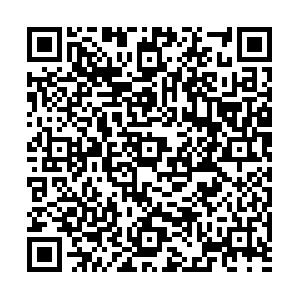# Discussions on the stability of diquarks

• Since the birth of the quark model, the diquark, which is composed of two quarks, has been considered as a substantial structure of a color anti-triplet. This is not only a mathematical simplification for dealing with baryons, but also provides a physical picture where the diquark would behave as a whole object. It is natural to ask whether such a structure is sufficiently stable against external disturbance. The mass spectra of the ground states of the scalar and axial-vector diquarks, which are composed of two-light (L-L), one-light-one-heavy (H-L) and two-heavy (H-H) quarks, respectively, have been calculated in terms of the QCD sum rules. We suggest a criterion as the quantitative standard for the stability of the diquark. It is the gap between the masses of the diquark and √s0 where s0 is the threshold of the excited states and continuity, namely the larger the gap is, the more stable the diquark would be. In this work, we calculate the masses of the H-H type to complete the series of the spectra of the ground state diquarks. However, as the criterion being taken, we find that all the gaps for the various diquarks are within a small range. In particular, the gap for the diquark with two heavy quarks, which is believed to be a stable structure, is slightly smaller than that of the other two types of diquarks. Therefore we conclude that because of the large theoretical uncertainty, we cannot use the numerical results obtained with the QCD sum rules to assess the stability of diquarks, but need to invoke other theoretical framework.
PCAS:
••Get Citation
TANG Liang and LI Xue-Qian. Discussions on the stability of diquarks[J]. Chinese Physics C, 2012, 36(7): 578-584. doi: 10.1088/1674-1137/36/7/002
TANG Liang and LI Xue-Qian. Discussions on the stability of diquarks[J]. Chinese Physics C, 2012, 36(7): 578-584.Milestone
Revised: 1900-01-01
Article Metric

Article Views(1703)
Cited by(0)
Policy on re-use
To reuse of subscription content published by CPC, the users need to request permission from CPC, unless the content was published under an Open Access license which automatically permits that type of reuse.
###### 通讯作者: 陈斌, bchen63@163.com
• 1.

沈阳化工大学材料科学与工程学院 沈阳 110142

Title:
Email:

## Discussions on the stability of diquarks

###### Corresponding author: LI Xue-Qian,

Abstract: Since the birth of the quark model, the diquark, which is composed of two quarks, has been considered as a substantial structure of a color anti-triplet. This is not only a mathematical simplification for dealing with baryons, but also provides a physical picture where the diquark would behave as a whole object. It is natural to ask whether such a structure is sufficiently stable against external disturbance. The mass spectra of the ground states of the scalar and axial-vector diquarks, which are composed of two-light (L-L), one-light-one-heavy (H-L) and two-heavy (H-H) quarks, respectively, have been calculated in terms of the QCD sum rules. We suggest a criterion as the quantitative standard for the stability of the diquark. It is the gap between the masses of the diquark and √s0 where s0 is the threshold of the excited states and continuity, namely the larger the gap is, the more stable the diquark would be. In this work, we calculate the masses of the H-H type to complete the series of the spectra of the ground state diquarks. However, as the criterion being taken, we find that all the gaps for the various diquarks are within a small range. In particular, the gap for the diquark with two heavy quarks, which is believed to be a stable structure, is slightly smaller than that of the other two types of diquarks. Therefore we conclude that because of the large theoretical uncertainty, we cannot use the numerical results obtained with the QCD sum rules to assess the stability of diquarks, but need to invoke other theoretical framework.

### HTML

Reference (1)
PDF查看关注分享

Top

### 目录/DownLoad:  Full-Size Img  PowerPoint